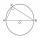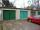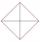# Right triangle - 9th grade (14y) - math problems

#### Number of problems found: 585

• Cube cutIn the ABCDA'B'C'D'cube, it is guided by the edge of the CC' a plane witch dividing the cube into two perpendicular four-sided and triangular prisms, whose volumes are 3:2. Determine in which ratio the edge AB is divided by this plane.
• CosineThe point (8, 6) is on the terminal side of angle θ. cos θ = ?
• DiagonalsDraw a square ABCD whose diagonals have a length of 6 cm
• Cone containerRotary cone-shaped container has a volume 1000 cubic cm and a height 12 cm. Calculate how much metal we need for making this package.
• SatinSanusha buys a piece of satin 2.4 m wide. The diagonal length of the fabric is 4m. What is the length of the piece of satin?
• The fenceI'm building a fence. Late is rounded up in semicircle. The tops of late in the field between the columns are to copy an imaginary circle. The tip of the first and last lath in the field is a circle whose radius is unknown. The length of the circle chord
• 3s prismIt is given a regular perpendicular triangular prism with a height 19.0 cm and a base edge length 7.1 cm. Calculate the volume of the prism.
• CircumscribingDetermine the radius of the circumscribed circle to the right triangle with legs 9 cm and 6 cm.
• Hexa prismDetermine the volume of hex prism with edge base 4 cm. The body height is 28 cm.
• ClimbFor horizontal distance 4.2 km road rise by 6.3 m. Calculate the road pitch in ‰ (permille, parts per thousand).
• Equilateral triangleA square is inscribed into an equilateral triangle with a side of 10 cm. Calculate the length of the square side.
• Isosceles trapezoidThe old father decided to change the top plate of an isosceles-like trapezoid with the basic dimensions of 120 cm and 60 cm, and the shoulder is 50 centimeters long. How much does it pay for a new plate and a square meter worth 17 euros?
• LathsThere are two laths in the garage opposite one another: one 2 meters long and the other 3 meters long. They fall against each other and lean against the opposite walls of the garage both laths and touch at a height of 70 cm above the garage floor. How wid
• Diagonal to areaCalculate the area of a rectangle in which the length of the diagonal is 10 cm.In the square ABCD point P is in the middle of the DC side and point Q in the middle pages AD. If the area of quadrilateral BQPC is 49 cm2, what is the area of ABCD?
• Circle tangentIt is given to a circle with the center S and radius 3.5 cm. Distance from the center to line p is 6 cm. Construct a circle tangent n which is perpendicular to the line p.
• TreeBetween points A and B is 50m. From A we see a tree at an angle 18°. From point B we see the tree in three times bigger angle. How tall is a tree?
• 30-60-90The longer leg of a 30°-60°-90° triangle measures 5. What is the length of the shorter leg?
• VectorCalculate length of the vector v⃗ = (9.75, 6.75, -6.5, -3.75, 2).
• Cone and the ratioRotational cone has a height 23 cm and the ratio of the base surface to lateral surface is 7: 9. Calculate a surface of the base and the lateral surface.

Do you have an interesting mathematical word problem that you can't solve it? Submit a math problem, and we can try to solve it.

We will send a solution to your e-mail address. Solved examples are also published here. Please enter the e-mail correctly and check whether you don't have a full mailbox.

Please do not submit problems from current active competitions such as Mathematical Olympiad, correspondence seminars etc...